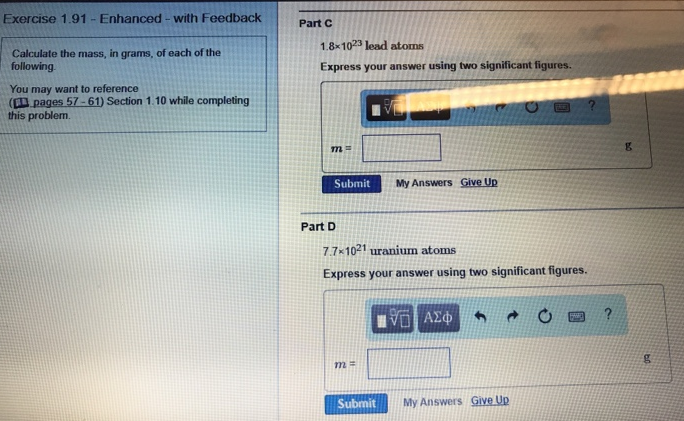# Problem: Calculate the mass, in grams, of each of the following. Part C1.8 x 1023 lead atomsExpress your answer using two significant figures.Part D7.7 x 1021 uranium atomsExpress your answer using two significant figures.

###### FREE Expert Solution
96% (96 ratings)###### Problem Details

Calculate the mass, in grams, of each of the following.

Part C

Part D

7.7 x 1021 uranium atoms